Next: FMRI data Up: Artificial data Previous: Methods

## Results

Figures 3--7 show the results of inferring on the three different continuous weights mixture models on the five different artificial datasets. The spatial maps in the figures are unthresholded marginal posterior means of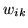, i.e., for all three classes of deactivation, non-activation and activation.

We can compute the effective global class proportions based upon the classifications, i.e.:(25)

where the weights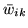are the mean marginal posterior weights. These effective global class proportions can be combined with the mean marginal posterior class distribution parameters to give a histogram fit. These are shown in figures 3--7(a).

The box plot in figure 8 shows the marginal posterior distributions of the MRF smoothness parameter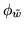for the different artificial datasets. The value ofclearly varies a lot from dataset to dataset emphasising the need for adaptive determination of the spatial smoothness.

It is interesting to compare model 2's performance with that of model 3. Model 3 is the same as model 2, except that in model 3 the spatial regularisation parameter is adaptive and in model 2 it is fixed. Model 2 works well on some datasets, for example figures 6 and 7. This is because the arbitrarily chosen value of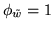is close to the adaptively determined values offor those datasets, which can be seen in figure 8 (note that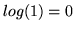). For the other datasets it works less well. For example, it overblurs the edges in figure 3 and overblurs the activation and deactivation to the point of removing much of it in figures 4 and 5.

It is worth emphasising that model 3 also works well on the no activation dataset (figure 7). This is a dataset where there is in fact only one class present (the non-activation class), and yet we fit the mixture model assuming three classes. Despite this, model 3 forces all probabilities to well less than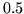for the activation and deactivation classes.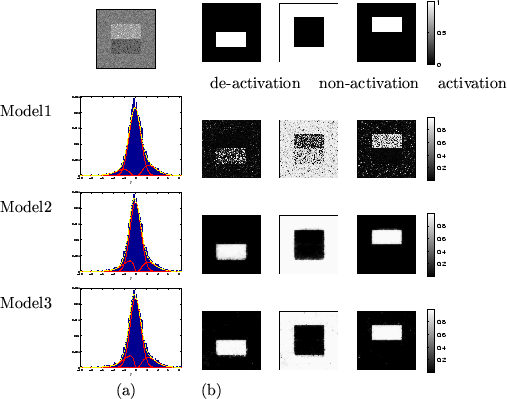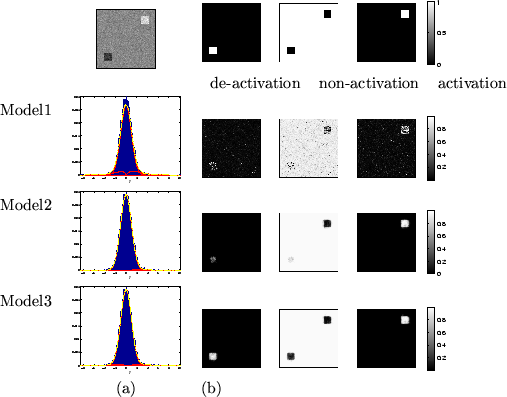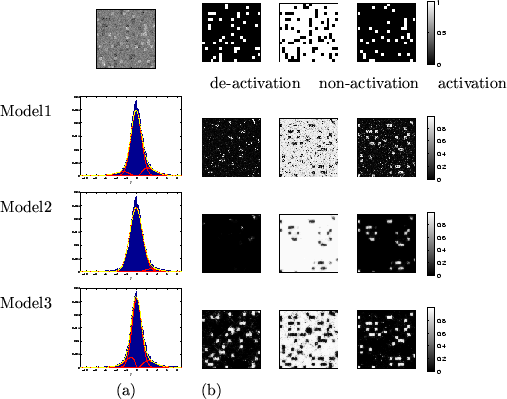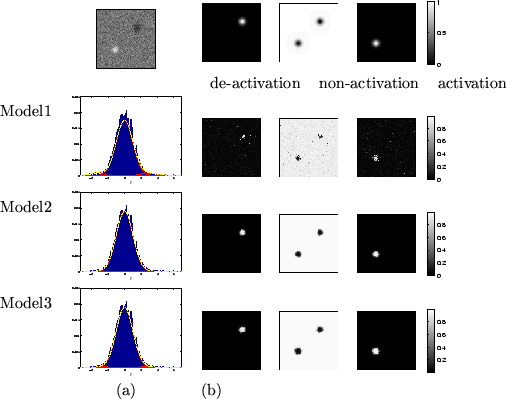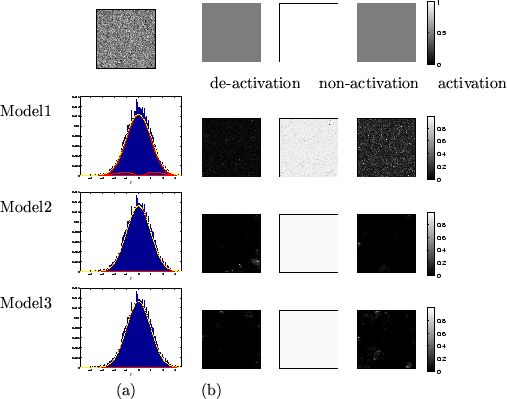Next: FMRI data Up: Artificial data Previous: Methods